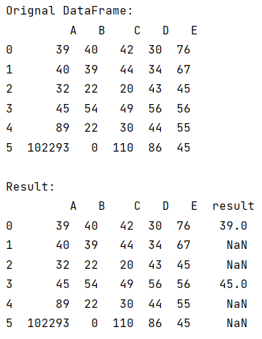# How to Compare Two Columns of Pandas DataFrame?

Given a Pandas DataFrame, we have to compare its two columns.
Submitted by Pranit Sharma, on June 22, 2022

Pandas is a special tool that allows us to perform complex manipulations of data effectively and efficiently. Inside pandas, we mostly deal with a dataset in the form of DataFrame. DataFrames are 2-dimensional data structures in pandas. DataFrames consists of rows, columns, and the data.

To compare two columns in pandas DataFrame, comparing columns is important as we need to understand the difference between the columns.
For this purpose, we will use a method called numpy.where(), it acts like a conditional statement that checks the condition defined inside it and then compares the columns.

Syntax:

```numpy.where(condition, [x, y, ]/)
```

To work with pandas, we need to import pandas package first, below is the syntax:

```import pandas as pd
```

Let us understand with the help of an example,

## Python Code to Compare Two Columns of Pandas DataFrame

```# Importing pandas
import pandas as pd

# Importing numpy package
import numpy as np

# Create a DataFrame
df = pd.DataFrame({
'A':[39,40,32,45,89,102293],
'B':[40,39,22,54,22,0],
'C':[42,44,20,49,30,110],
'D':[30,34,43,56,44,86],
'E':[76,67,45,56,55,45]
})

# Display original DataFrame
print("Orignal DataFrame:\n",df,"\n")

# Comparing two columns
df['result'] = np.where((df['A'] <= df['B']) & (
df['A'] <= df['C']), df['A'], np.nan)

# Display result
print("Result:\n",df)
```

Output:Languages: » C » C++ » C++ STL » Java » Data Structure » C#.Net » Android » Kotlin » SQL
Web Technologies: » PHP » Python » JavaScript » CSS » Ajax » Node.js » Web programming/HTML
Solved programs: » C » C++ » DS » Java » C#
Aptitude que. & ans.: » C » C++ » Java » DBMS
Interview que. & ans.: » C » Embedded C » Java » SEO » HR
CS Subjects: » CS Basics » O.S. » Networks » DBMS » Embedded Systems » Cloud Computing
» Machine learning » CS Organizations » Linux » DOS
More: » Articles » Puzzles » News/Updates